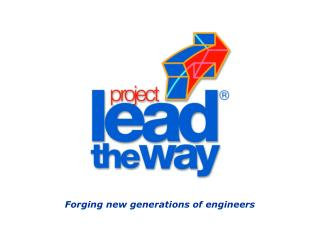# Forging new generations of engineers - PowerPoint PPT PresentationDownload PresentationForging new generations of engineers

Forging new generations of engineersDownload Presentation## Forging new generations of engineers

- - - - - - - - - - - - - - - - - - - - - - - - - - - E N D - - - - - - - - - - - - - - - - - - - - - - - - - - -
##### Presentation Transcript

1. Forging new generations of engineers

2. Vector Calculations

3. Vectors A force is often shown as a vector. A force that is not parallel to the x or y axis can be broken up into its x and y components. (The arrow symbol indicates that force, F, is a vector.)

4. Fx Fy Vectors is the x-component of force, F. is the y-component of force, F.

5. Vectors • If we know • the angle, , of F from an axis, and • the magnitude of F, • we can further define its components.

6. Vectors Notice the arrow-symbol does not appear above the “F” in these formulas. This indicates that we are solving for the magnitudes (the scalar quantities only) of these forces. Fx = F * cos  Fy = F * sin 

7. y - axis 100 lbs 30 x - axis Vectors This example, F is 100lbs, and  is 30.

8. y - axis 100 lbs 30 x - axis Vectors Fx = F * cos  Fx = 100lbs * cos 30 Fx = 87 lbs Fy = F * sin  Fy = 100lbs * sin 30 Fy = 50 lbs The x-component of F is a vector. Its magnitude is 87 lbs, and it’s direction is the x-direction. The y-component of F is also vector. Its magnitude is 50 lbs, and it’s direction is the y-direction.

9. Vectors Vectors can be combined. The resultant force is the result of adding or subtracting vectors. Here, vectors F1 and F2 are being graphically added together. The resultant vector will be called F3.

10. Vectors To add the vectors graphically, place them head to tail (F2 first, then F1 -The head of the vector is the end with the arrow head). Draw the resultant vector from the first tail to the last head. Try starting with F1 instead. Is the resultant vector different?

11. F2 F2=100 lb F1=50 lb F1 F3y F3x After you draw the resultant force, F3, find the magnitudes of its x and y components by measuring them against the axes. Vectors To solve a vector problem using graph paper, label an x and y axis and label a scale along each axis. For example: y - axis y - axis 100 80 60 40 20 F3 x - axis x - axis 0 20 40 60 80 100

12. y - axis F2=100 lb F1=50 lb 45 30  x - axis Vectors In this example, F1 and F2 are being mathematically added together. The resultant vector will be called F3.

13. Find the x and y components of vector F2 : F2x = F2 * cos 2 F2x = 100 lbs * cos 30 F2x = 87 lbs x-components of each Vectors In this example, F1 = 50 lbs at an angle of 45 degrees and, F2 = 100 lbs at an angle of 30 degrees. Find the x and y components of vector F1 : F1x = F1 * cos 1 F1x = 50 lbs * cos 45 F1x = 35 lbs

14. Find the x and y components of vector F2 : F2y = F2 * sin 2 F2y = 100 lbs * sin 30 F2y = 50 lbs y-components of each Vectors In this example, F1 = 50 lbs at an angle of 45 degrees and, F2 = 100 lbs at an angle of 30 degrees. Find the x and y components of vector F1 : F1y = F1 * sin 1 F1y = 50 lbs * sin 45 F1y = 35 lbs

15. Vectors In this example, F1 = 50 lbs at an angle of 45 degrees and, F2 = 100 lbs at an angle of 30 degrees. Now, find the x and y components of the resultant vector. To do this add the x components together, then the y components together.

16. Vectors